# Machine Learning Model for Stochastic Processes

## Prediction of Loan Status Using Monte Carlo Simulation

Abstract: Using the loan_timing.csv dataset provided, we built a simple model using the Monte Carlo simulation for predicting the fraction of loans that will default after the 3-year duration of the loan. Our model revealed a 95% confidence interval of 14.8% +/- 0.2% for Monte-Carlo simulation of N = 1000 replicated copies of the dataset. Based on these analyses, if 50,000 loans were given out with a loan term of 3 years, approximately 15% of these loans will default during the loan term.

Introduction: Predicting the status of a loan is an important problem in risk assessment. A bank or financial organization has to be able to estimate the risk involved before granting a loan to a customer. Data Science and predictive analytics play an important role in building models that can be used for predicting the probability of loan default. In this project, we are provided with the loan_timing.csv dataset containing 50000 data points. Each data point represents a loan, and two features are provided as follows:

1. The column with header “days since origination” indicates the number of days that elapsed between origination and the date when the data was collected.
2. For loans that charged off before the data was collected, the column with header “days from origination to charge-off” indicates the number of days that elapsed between origination and charge-off. For all other loans, this column is blank.

Definition of Technical Terms

1. Origination: This refers to the date when borrower receives a loan from a lender.
2. Charge-off (loan default) Status: The borrower then makes regular repayments, until the borrower stops making payments, typically due to financial hardship, before the end of the loan term. This event is called charge-off, and the loan is then said to have charged off or in default state.
3. Current or Active Status: The borrower continues making repayments over the entire loan term. At this point, the debt has been fully repaid.
4. Loan Term: Period over which a loan agreement is in force, and before or at the end of which the loan should either be repaid or renegotiated for another term. In this example, we consider a loan with a term or duration of 3 years.

Project Objective: The goal of this project is to use techniques of data science to estimate what fraction of these loans (50,000 customer records in the loan_timing.csv dataset) will have charged off during the 3-year loan term.

The dataset and R code for this article can be downloaded from this repository: https://github.com/bot13956/Monte_Carlo_Simulation_Loan_Status.

# Model Implementation Using R

Import Necessary Libraries

`library(readr)library(tidyverse)library(broom)library(caret)`

Import Dataset

`df<-read_csv("loan_timing.csv",na="NA")names(df)=c("origination","chargeoff")# partition data set into two: default (charged off ) and currentindex<-which(!(df\$chargeoff=="NA"))default<-df%>%slice(index)current<-df%>%slice(-index)`

Exploratory Data Analysis

`# Figure 1: Histogram of days since origination for current loanscurrent%>%ggplot(aes(origination))+geom_histogram(color="white",fill="skyblue")+xlab('days since origination')+ylab('count')+ggtitle("Histogram of days since origination for current loans")+theme(plot.title = element_text(color="black", size=12, hjust=0.5, face="bold"),axis.title.x = element_text(color="black", size=12, face="bold"),axis.title.y = element_text(color="black", size=12, face="bold"),legend.title = element_blank())`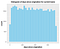Figure 1: Histogram of days since origination for current loans.
`# Figure 2: Histogram of days to charge-off for defaulted loansdefault%>%ggplot(aes(chargeoff))+geom_histogram(color="white",fill="skyblue")+xlab('days to charge-off')+ylab('count')+ggtitle("Histogram of days to charge-off for defaulted loans")+theme(plot.title = element_text(color="black", size=12, hjust=0.5, face="bold"),axis.title.x = element_text(color="black", size=12, face="bold"),axis.title.y = element_text(color="black", size=12, face="bold"),legend.title = element_blank())`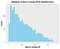Figure 2: Histogram of days to charge-off for defaulted loans.
`# Figure 3: Histogram of days since origination for defaulted loansdefault%>%ggplot(aes(origination))+geom_histogram(color="white",fill="skyblue")+xlab('days since origination')+ylab('count')+ggtitle("Histogram of days since origination for defaulted loans")+theme(plot.title = element_text(color="black", size=12, hjust=0.5, face="bold"),axis.title.x = element_text(color="black", size=12, face="bold"),axis.title.y = element_text(color="black", size=12, face="bold"),legend.title = element_blank())`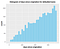Figure 3: Histogram of days since origination for defaulted loans.

Figure 1 shows the histogram of active loans, which are uniformly distributed over the days since origination.

From Figure 2, we see that the proportion of loans that charged off decreases with increasing days from origination to charge-off. This shows that younger loans have a higher probability of default. It also shows that 100% of loans defaulted within 2 years from the date of origination.

Figure 3 shows the distribution of defaulted loans as a function of days since origination to the time when data about loan status was collected. The defaulted loans contain a large proportion (71%) of loans that are one year and older. These loans are less likely to default compared to younger loans.

`# Figure 4: Plot of days to charge-off vs. days since origination for defaulted loansdefault%>%ggplot(aes(origination,chargeoff))+geom_point()+xlab('days since origination')+ylab('days to charge-off')+ggtitle("days to charge-off vs. days since origination")+theme(plot.title = element_text(color="black", size=12, hjust=0.5, face="bold"),axis.title.x = element_text(color="black", size=12, face="bold"),axis.title.y = element_text(color="black", size=12, face="bold"),legend.title = element_blank())`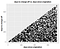Figure 4: Plot of days to charge-off vs. days since origination for defaulted loans.

Model Selection: Our dataset has only 2 features or predictors, and suffers from the problem of prevalence: 93% of the loans have an active status, while 7% have a default status. Use of Linear Regression for predicting the fraction of loans that will have charged off after the 3 years loan duration produces a model that is biased towards the active loans.

Figure 4 indicates the relationship between days to charge-off and days since origination for defaulted loans can be simulated using Monte Carlo (MC) simulation. We, therefore, choose MC simulation as our model for the predictive proportion of loans that will default.

Model Calculations: We generated an MC simulation of the defaulted loans, and compared it with the original data.

`# Monte Carlo Simulation of Defaulted Loansset.seed(2)N <- 3*365 # loan duration in daysdf_MC<-data.frame(u=round(runif(15500,0,N)),v=round(runif(15500,0,N)))df_MC<-df_MC%>%filter(v<=u)df_MC<-df_MC%>%filter(u<=730 & v<=730) #select loans within first 2 yearsdf_MC[1:nrow(default),]%>%ggplot(aes(u,v))+geom_point()+xlab('days since origination')+ylab('days to charge-off')+ggtitle("MC simulation of days to charge-off vs. days since origination")+theme(plot.title = element_text(color="black", size=12, hjust=0.5, face="bold"),axis.title.x = element_text(color="black", size=12, face="bold"),axis.title.y = element_text(color="black", size=12, face="bold"),legend.title = element_blank())`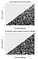Figure 5: Original and MC simulation of days to charge-off vs. days since origination.

Because there is randomness associated with the charge-off of a loan, we see that MC simulation provides a good approximation for the distribution of defaulted loans.

Predictions: Since we have demonstrated that the relationship between days to charge-off and days since origination in the first 2 years (i.e. 0 to 730 days) can be approximated using an MC simulation, we can predict the fraction of loans that will be charged off by the time all of their 3-year terms are finished using MC simulation.

The total number of charged-off loans in our dataset is 3,305. This means that there are 46,695 loans that are currently active. Of these active loans, a certain proportion will default over the 3-year period. To estimate the total fraction of defaulted loans, we simulated defaulted loans with charge-off and days since origination covering the entire duration of the loan (i.e. 0 to 1095 days), then by appropriate scaling, we computed the fraction of loans that will have charged off after the 3-year term i.e., 1095 days.

`# Predicting fraction of these loans will have charged off by the time all of their 3-year term is finished.set.seed(2)B<-1000fraction<-replicate(B, {df2<-data.frame(u=round(runif(50000,0,N)),v=round(runif(50000,0,N)))df2<-df2%>%filter(v<=u)b2<-(df2%>%filter(u<=730 & v<=730))total<-(nrow(df2)/nrow(b2))*nrow(default)100.0*(total/50000.0)})mean(fraction)# Histogram of total fraction of charged off loansfdf<-data.frame(fraction=fraction)fdf%>%ggplot(aes(fraction))+geom_histogram(color="white",fill="skyblue")+xlab('fraction of charged off loans after 3-year term')+ylab('count')+ggtitle("Histogram of total fraction of charged off loans")+theme(plot.title = element_text(color="black", size=12, hjust=0.5, face="bold"),axis.title.x = element_text(color="black", size=12, face="bold"),axis.title.y = element_text(color="black", size=12, face="bold"),legend.title = element_blank())# Calculate Confidence Interval of Percentage of Defaulted Loans after 3-year termmean<-mean(fraction)sd<-sd(fraction)confidence_interval<-c(mean-2*sd, mean+2*sd)confidence_interval`

By creating 1000 random trials, we obtained the following distribution for the fraction of defaulted loans 3-year term: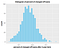Figure 6: Histogram for fraction of charged-off loans after 3-year term using N = 1000 samples.

Based on our calculations, the 95% confidence interval for the fraction of loans that will have charged off after the 3-year loan duration is accordingly 14.8% +/- 0.2%. So if 50,000 loans were given out with a loan term of 3 years, approximately 15% of these loans will default.

Conclusions: We have presented a simple model based on the MC simulation for predicting the fraction of loans that will default at the end of the 3-year loan duration period. Monte Carlo simulation is an important method that can be used in prescriptive analytics for the prescribing course of action to be taken in cases where the dataset is very stochastic in nature.

The dataset and R code for this article can be downloaded from this repository: https://github.com/bot13956/Monte_Carlo_Simulation_Loan_Status.

Written by

## Towards AI

#### Towards AI, is the world’s fastest-growing AI community for learning, programming, building and implementing AI.

Welcome to a place where words matter. On Medium, smart voices and original ideas take center stage - with no ads in sight. Watch
Follow all the topics you care about, and we’ll deliver the best stories for you to your homepage and inbox. Explore
Get unlimited access to the best stories on Medium — and support writers while you’re at it. Just \$5/month. Upgrade